# A New Hybrid Viscoelastic Soft Tissue Model based on Meshless Method for Haptic Surgical Simulation

Yidong Baoa, b, Dongmei Wua, *, Zhiyuan Yan a, Zhijiang Dua
a State Key Laboratory of Robotics and System, Harbin Institute of Technology, Harbin 150080, P.R. China
b School of Software, Pingdingshan University, Pingdingshan 467000, P.R. China

#### Article Metrics

0
##### Total Statistics:

Full-Text HTML Views: 6479
Abstract HTML Views: 1197
##### Unique Statistics:

Full-Text HTML Views: 1346
Abstract HTML Views: 658

open-access license: This is an open access article licensed under the terms of the Creative Commons Attribution Non-Commercial License (http://creativecommons.org/licenses/by-nc/3.0/) which permits unrestricted, non-commercial use, distribution and reproduction in any medium, provided the work is properly cited.

* Address correspondence to this author at the State Key Laboratory of Robotics and System, Harbin Institute of Technology, Harbin 150080, China; Tel: +86 0451-86414462-18; Fax: +86 0451-86414174; E-mail: wudmhit@163.com

## Abstract

This paper proposes a hybrid soft tissue model that consists of a multilayer structure and many spheres for surgical simulation system based on meshless. To improve accuracy of the model, tension is added to the three-parameter viscoelastic structure that connects the two spheres. By using haptic device, the three-parameter viscoelastic model (TPM) produces accurate deformationand also has better stress-strain, stress relaxation and creep properties. Stress relaxation and creep formulas have been obtained by mathematical formula derivation. Comparing with the experimental results of the real pig liver which were reported by Evren et al. and Amy et al., the curve lines of stress-strain, stress relaxation and creep of TPM are close to the experimental data of the real liver. Simulated results show that TPM has better real-time, stability and accuracy.

Keywords: : Meshless, soft tissue model, force-feedback, viscoelasticity , haptic device, surgical simulation..

## 1. INTRODUCTION

Virtual surgery simulation enables physicians to interact with flexibly customized simulate environments based on visual, auditory and haptic feedback without potentially harmful contact with real patients. With the use of virtual surgery, surgeons have the opportunity to surgically train in a low-cost and ethically sound environment . On the other hand, real-time surgical simulation and medical training must employ the physical modeling and behavioral modeling of soft tissue so as to achieve realistic visual and tactile effects .

Deformable models can be classified into two categories: physics-based and geometry-based. Physics-based methods are based on continuum mechanics, and can get accurate simulation results by directly solving the partial differential equations (PDEs) using numerical methods. Some of the prevailing methods include the finite element method (FEM), mass-spring model (MSM), boundary element method (BEM), point-based method. Geometry-based models use intuitive methods instead of solving PDE. For example, vertex based model and spline based model. The advantages of geometry-based approaches are: fast and smooth, but the major drawback is in their accuracy. In contrast, physics-model is more accurate than geometry based approaches, but it has the disadvantages of being slow and rough. The key point to build a physical model of soft tissue is to reduce computational complexity and simultaneously keep the accuracy of deformation effects .

A material is called viscoelasticity if the stress–strain relationship of the material depends on time under applied forces. Ratchada  applied the method of tissue creep and stress relaxation to parallel-fibered collagenous tissues. Cotin  established a hybrid elastic model for surgery training and simulation. Suzuki  created a deformable organ model by using sphere-filled method instead of the finite element method. But the deformation of the model is still based on MSM. Recently, meshless methods have been introduced in the computer graphics field for a real-time simulation of deformable objects. The meshless method, in contrast to the mesh-based method, discretized the object by merely using a set of nodes without a mesh structure. This method thus avoided the problems associated with remeshing and handles the topology change of the object in a relatively simple manner. The topological relation among meshless simulation nodes is defined by support of the node. Adjacent nodes, which lie within the support, acted like the nodes which are connected by the edges of the mesh in the mesh-based method . Then researchers start to set up the meshless physical model such as potential energy method , discrete mechanics , which has become a popular research in soft tissue modeling and cutting.

Haptic feedback is especially important in delicate surgical pressures requiring fine sensations, especially when slightly excessive pressure can injure a patient. The ability to touch the virtual objects in the environments is one factor that provides a more realistic experience and is particularly suited to virtual training applications . So, the create process is related to the constraint force needed to keep a valid model which is close to the real tissue from the relaxation and creep and other properties.

## 2. MODEL STRUCTURE

### 2.1 . Layered Sphere-Filled Structure

A multilayer structure has been adopted to arrange the TPM, as shown in Fig. (1, a). The TPM has 424 spheres and the radius of the sphere is 10mm. These spheres are connected by a three-parameter structure which is composed of a Kelvin model and a linear spring.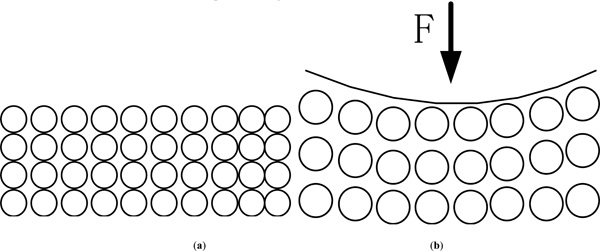Fig. (1). (a) Layered sphere-filled structure. (b) The structure of layered sphere-filled model under the action of force.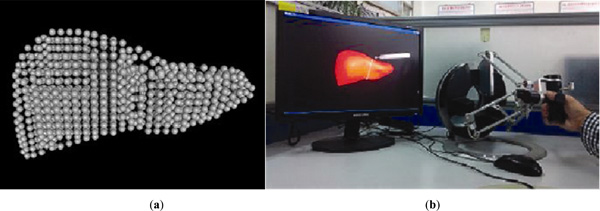Fig. (2). (a)The structure of layered sphere-filled based on meshless method in TPM model has been established by using VC++ and the OpenGL. (b) Our test platform with a PC and a Omega.7.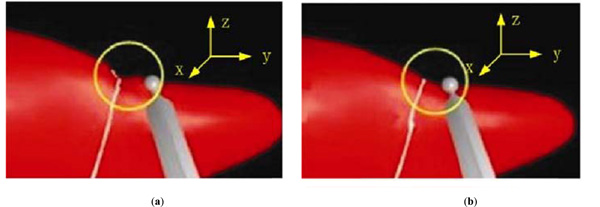Fig. (3). (a) Loading 2N in the Z-axis positive direction, the liver model based on MSM presents the deformation rendering. (b) Loading 2N in the Z-axis positive direction, the liver model based on meshless method TPM model presents the deformation rendering.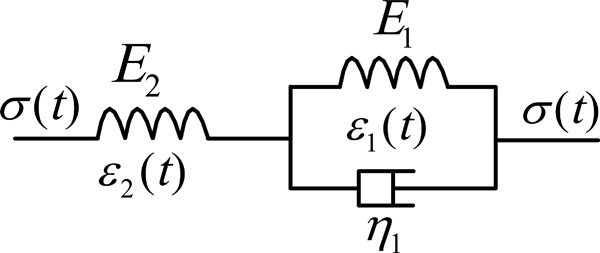Fig. (4). Three-parameter viscoelastic structure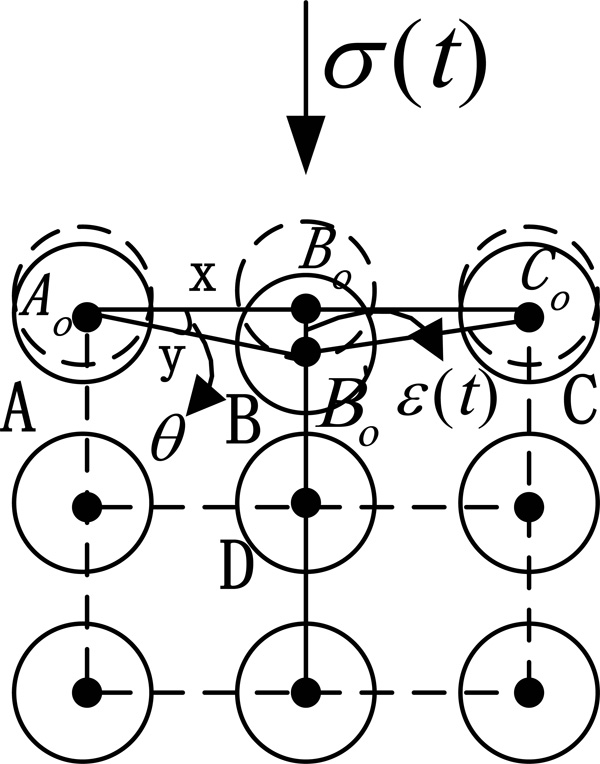Fig. (5). The stress status of TPM model when exert the external stress to outer sphere.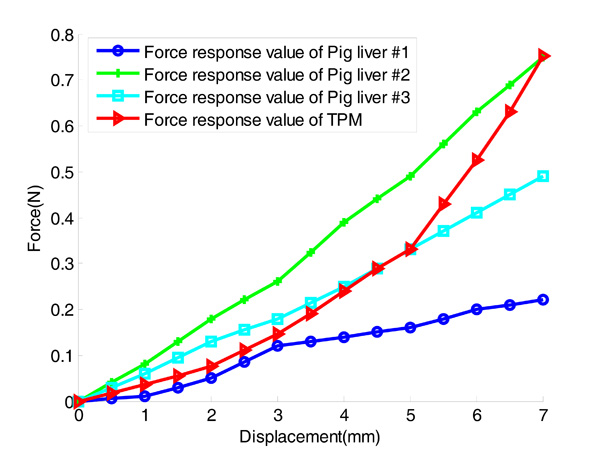Fig. (6). The comparison between the force response of experimental data of three pig livers and the force response of TPM model.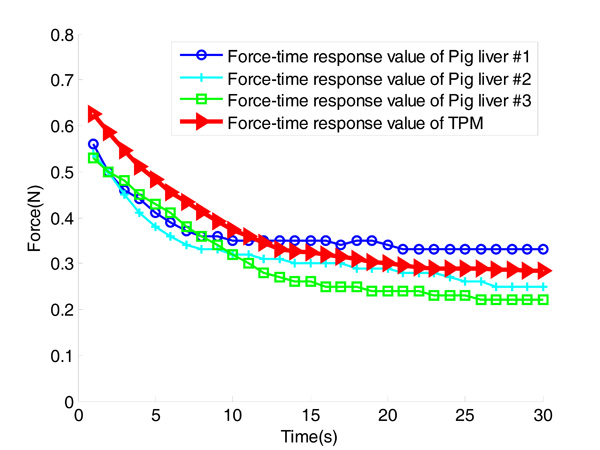Fig. (7). The comparison between the force-time response of experimental data of three pig livers and the force-time response of TPM model for the indentation depth of 4 mm with a strain rate of 4mm/s in 30 seconds.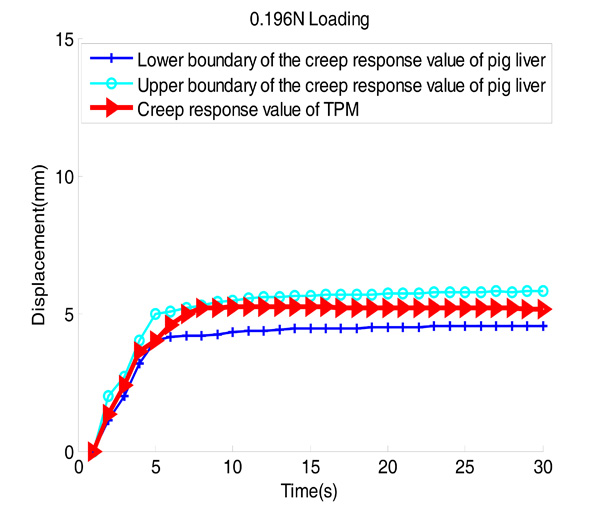Fig. (8). The comparison between the creep response of Ex vivo perfused pig liver loading 0.196N in the Z-axis positive direction and the creep response of TPM model.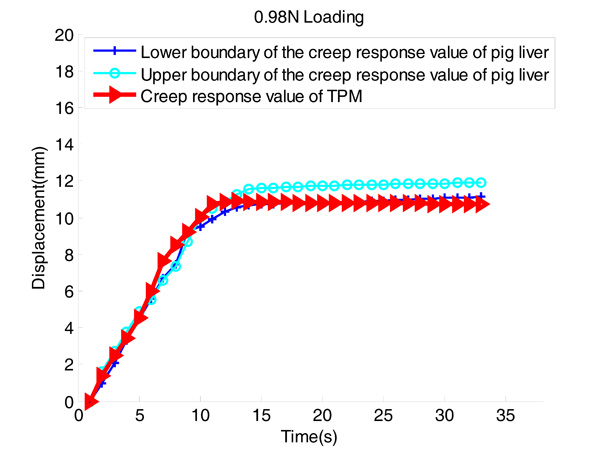Fig. (9). The comparison between the creep response of Ex vivo perfused pig liver loading 0.98N in the Z-axis positive direction and the creep response of TPM model.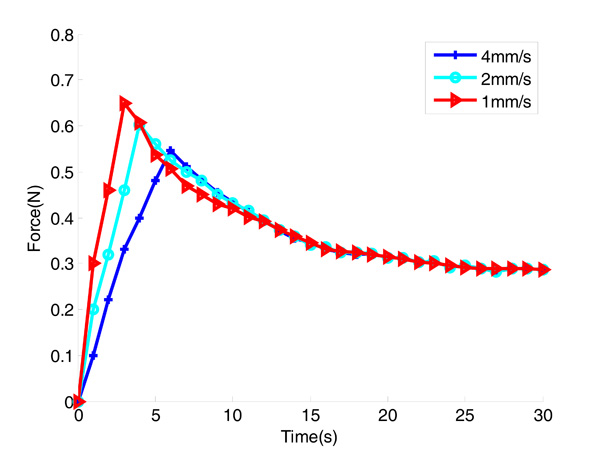Fig. (10). The relaxation curve recorded at the indentation depth of 4 mm at strain rates of 4, 2, and 1 mm/s in TPM model.

Under external force, the deformation of the outer-layer sphere is obvious, and the deformation of the inner-layer sphere will weaken orderly. The deformation effects fit the deformation of the real soft tissue, which is shown in Fig. (1, b). Fig. (2, a) presents the structure of layered sphere-filled based on meshless method in TPM. This model has been established by using VC++ and the OpenGL.

To clarify this point further, the liver tissue model has been established by using MSM structure in this paper. Loading 2 newtons (N) in the Z-axis positive direction which act on the MSM, the deformation effects of the liver model are shown in Fig. (3, a). Similarly, loading 2N in the Z-axis positive direction which act on TPM, the deformation effects of the liver model are shown in Fig. (3, b). In the MSM model, the deformation effects of the force point and edges are roughness, as shown in Fig. (3, a, b). But TPM model has better effects in deformation, especially the deformation of the force point and edges.

### 2.2 . Real-Time Simulation Condition

We used the hardware platforms that included Omega.7 force-feedback devices which came from the Swiss Force Dimension Company, a computer which consisted of AMD Athlon 64X2 2.0GHz CPU 2.0GB RAM and Samsung LCD, as shown in Fig. (2, b). The graphic interfaces OpenGL can be used to display the effect of graphics rendering and visualization on this model simulation program. Programs of virtual scene setting and force-feedback effect came from the open source programs of the Swiss Force Dimension Company (http://www.chai3d.org /download.html).

## 3. MODEL FORMULATION

This model consisted of tension and three-parameter viscoelastic structure  which had creep effects and viscoelastic properties. Finally, stress relaxation and creep formulas had been obtained by mathematical formula derivation.

### 3.1 . Model Framework

A three-parameter viscoelastic structure consists of a Kelvin model and a spring as the basic model was used in this paper, as shown in Fig. (4). In Fig. (4), we assume that ${\sigma }_{h}\left(t\right)$ is the stress of three-parameter structure of two nodes at t time, E1 and E1 are the elastic modulus of the spring, η1 is the viscous modulus, ${\epsilon }_{1}\left(t\right)\mathrm{and}{\epsilon }_{2}\left(t\right)$ are the length of the spring at time.

It will be damaged when the deformation length of the liver is more than 12mm  by using a rigid object to touch the liver surface with different velocity. So, the deformation length of TPM model should remain within 10mm as an effective data by using a rigid sphere with a radius of 2mm to touch the model. Here, we set the radius of the sphere in TPM model as 10mm when exert external stress $\sigma \left(t\right)$ is exerted to the outer sphere in this model, the stress status as shown in Fig. (5). It can be seen from Fig. (5) that the displacement of the outer sphere B changed under stress. To ensure the validity of the deformation, the displacement of sphere B should remain within 10mm. In order to simplify the calculation, we ignore the displacement of the spheres A and C. Here, we assume that ${A}_{o}$ is the center of the sphere A. Similarly, ${B}_{o},{C}_{o},{D}_{o}$ are the centers of spheres B, C, D, respectively. And ${B}_{o}^{\text{'}}$ is the center of the sphere B after deformation between two spheres which are connected by a three-parameter viscoelastic structure.

We assume that $\epsilon \left(t\right)$ is the distance between ${B}_{o}$ and ${B}_{o}^{\text{'}}$. The initial length of line AB is x, and the length of line AB is y after deformation. The angle between x and y is. Then, we assume $\Delta \epsilon \left(t\right)=y-x$. The length of deformation between A and B can be defined as

(1)  $\left(t\right)=\left(t\right)\left(\frac{1\mathrm{cos}}{\mathrm{sin}}\right)$

The deformation length of this model should remain within 12mm to avoid the model damage. In order to ensure this criterion, θ should satisfy that $\theta \in \left(0-\pi /6\right)$. Here, we assume that ${\sigma }_{f}\left(t\right)$ is the tension. Then, the tension can be defined as

(2)  ${}_{f}\left(t\right)=2{E}^{\text{'}}\left(t\right)\left(\frac{1\mathrm{cos}}{\mathrm{sin}}\right)\mathrm{sin}$

where E' is the elastic modular.We assume ${\lambda }_{1}=2{E}^{\text{'}}\left(1-\mathrm{cos}\theta \right)$, then Eq.(2) can be written as ${\sigma }_{f}\left(t\right)={\lambda }_{1}\cdot \epsilon \left(t\right)$.

The formula of this model includes:

(3)  $\left(t\right)={}_{1}\left(t\right){}_{2}\left(t\right)$

(4)  ${}_{h}\left(t\right)={E}_{1}{}_{1}\left(t\right){}_{1}{\stackrel{}{}}_{1}\left(t\right)$

(5)  ${}_{h}\left(t\right)={E}_{2}{}_{2}\left(t\right)$

(6)  ${}_{f}\left(t\right)={}_{1}\left(t\right)$

(7)  $\left(t\right)={}_{h}\left(t\right){}_{f}\left(t\right)$

By using inverse Laplace transform, we can obtain

(8)  $\begin{array}{c}{E}_{1}{E}_{2}\left(t\right){E}_{2}{}_{1}\stackrel{}{}\left(t\right){}_{1}\left({E}_{1}{E}_{2}\right)\left(t\right){}_{1}{}_{1}\stackrel{}{}\left(t\right)=\\ \left({E}_{1}{E}_{2}\right)\left(t\right){}_{1}\stackrel{}{}\left(t\right)\end{array}$

This assumes ${P}_{1}=\frac{{}_{1}}{{E}_{1}{E}_{2}}$, ${P}_{2}=\frac{{E}_{1}{E}_{2}}{{E}_{1}+{E}_{2}}$, ${P}_{3}=\frac{{\eta }_{1}{E}_{2}}{{E}_{1}+{E}_{2}}$, Eq.(8) can be written as

(9)  $\left({P}_{2}{}_{1}\right)\left(t\right)\left({P}_{3}{}_{1}{P}_{1}\right)\stackrel{}{}\left(t\right)=\left(t\right){P}_{1}\stackrel{}{}\left(t\right)$

Eq.(9) is the constitutive equation of this model.

### 3.2 . Relaxation

After noting that $\epsilon \left(t\right)={\epsilon }_{0}H\left(t\right)$. Here, $H\left(t\right)$ is the unit step function. It can be defined as

By using Laplace transform , Eq.(9) can be written as

(10)  $\stackrel{}{}\left(s\right)=\frac{{}_{0}}{{P}_{1}}\left[\frac{{P}_{3}{P}_{1}{P}_{2}}{\frac{1}{{P}_{1}}s}\frac{{P}_{1}\left({P}_{2}{}_{1}\right)}{s}\right]$

By using inverse Laplace transform, Eq.(10) can be written as

(11)  $\left(t\right)=\left({E}_{2}{}_{1}\right){}_{0}\frac{{E}_{2}^{2}{}_{0}}{{E}_{1}{E}_{2}}\left(1{e}^{\frac{t\left({E}_{1}{E}_{2}\right)}{{}_{1}}}\right)$

Eq.(11) is the relaxation equation of this model.

### 3.3 . Creep

To exert a force ${\sigma }_{0}$ on soft tissue, there is a step-response function $H\left(t\right)$. Considering load $\sigma \left(t\right)={\sigma }_{0}H\left(t\right)$, substituting $\overline{\sigma }\left(t\right)={\sigma }_{0}/S$ and $\overline{\stackrel{˙}{\sigma }}\left(t\right)=S\overline{\sigma }\left(t\right)={\sigma }_{0}$ into Eq.(9), by using Laplace transform, Eq.(9) can be written as

(12)  $\frac{{}_{0}}{s}{P}_{1}{}_{0}=\left({P}_{2}{}_{1}\right)\stackrel{}{}\left(s\right)\left({P}_{3}{}_{1}{P}_{1}\right)s\stackrel{}{}\left(s\right)$

Eq.(12) can be written as

(13)  $\begin{array}{c}\stackrel{}{}\left(s\right)=\frac{{}_{0}}{S}\left(\frac{1{P}_{1}S}{\left({P}_{2}{}_{1}\right)\left({P}_{3}{}_{1}{P}_{1}\right)S}\right)=\frac{{}_{0}}{{P}_{3}{}_{1}{P}_{1}}\\ \left[\frac{1}{S\left(S\frac{\left({P}_{2}{}_{1}\right)}{\left({P}_{3}{}_{1}{P}_{1}\right)}\right)}\frac{{P}_{1}}{S\frac{\left({P}_{2}{}_{1}\right)}{\left({P}_{3}{}_{1}{P}_{1}\right)}}\right]\end{array}$

This assumes $\frac{{P}_{2}+{\lambda }_{1}}{{P}_{3}+{\lambda }_{1}{P}_{1}}=\frac{1}{{\tau }_{1}}$, by using inverse Laplace transform, Eq.(13) can be written as

(14)  $\left(t\right)=\frac{{}_{0}}{{P}_{3}{}_{1}{P}_{1}}\left[{}_{1}\left(1{e}^{\frac{t}{{}_{1}}}\right){P}_{1}{e}^{\frac{t}{{}_{1}}}\right]$

Eq.(14) is the creep equation of this model.

Eq.(14) shows that the sphere will be stable under the constant force ${\sigma }_{0}$ after t time, then the displacement of this sphere is $\mathrm{\epsilon \left(t\right)}$. The relationship between ${\sigma }_{0}$ and $\mathrm{\epsilon \left(t\right)}$ is the stress-strain in this model. The displacement ε is changed with time t that can be defined as creep. Eq.(11) shows that the force will change with the time when we give it a constant displacement ${\epsilon }_{0}$. The force $\mathrm{\sigma \left(t\right)}$ is changed with time t that can be defined as relaxation.

## 4. RESULTS

### 4.1 . Model Parameters

The effective elastic modulus of soft organ was estimated from the force versus displacement data of static indentations based on the small deformation assumption. Carter  and Kauer  used an instrument manually to measure the displacement and force response of the organ surface. In experiments with ex vivo pig liver, by using a hand-held probe Carter  calculated that the elastic modulus of pig liver which was equal to 490 kPa . Ottensmeyer  designed a robotic indenter to measure mechanical properties of pig liver during a minimally invasive surgery. The robotic indenter can make small indentations in the range of ±500 µm. In experiments with in vivo pig liver, he calculated that the elastic modulus of pig liver was equal to 2.2–15 kPa.

Effective shear and elastic moduli of pig liver were estimated from the static indentation data by using the linear elastic contact theory and the small deformation assumption. The effective shear modulus, G, for small indentation of an elastic half space by a rigid hemispherical indenter can be obtained using the solution given by Lee . So, by using the method of Lee the effective elastic modulus value was calculated between 11.6-18.7kPa . On the other hand, in order to achieve better results, compared with the hand-held instrument, a robotic indenter was developed for minimally invasive measurement of the tissue properties. So we selected the parameter that was published by Ottensmeyer. Then we used the elastic modulus value of the liver which was equal to 12 kPa in this paper by combining these parameters.

It can be seen from Fig. (5), under static stress ${\sigma }_{0}$ loading, we have $\left(t\right)={}_{1}\left(t\right){}_{2}\left(t\right)$ and ${\sigma }_{0}={E}_{1}{\epsilon }_{1}\left(t\right)+{E}_{2}{\epsilon }_{2}\left(t\right)$. Then, we can write that ${\sigma }_{0}={E}^{\text{'}}\epsilon \left(t\right)$.Therefore, we get that ${E}^{\text{'}}={E}_{1}={E}_{2}$=12kPa. For ${\lambda }_{1}=2{E}^{\text{'}}\left(1-\mathrm{cos}\theta \right)$,$\theta \in \left(0-\pi /4\right)$, the value of ${\lambda }_{1}$ is calculated between 0.096kPa and 5.9kPa.

Klatt  had concluded that the best agreement between fit and experiment was achieved with the Zener model. The reported value for viscous modulus base on Zener viscoelastic model was equal to 5.5 ± 1.6 Pa.s. Mohammad  calculated that the viscous modulus value was between 0.8-1.57 Pa.s. Asbach  calculated that the viscous modulus value was equal to 5Pa.s. This deviation between our modeling results and other experiment results not only because of the model type (Voigt or Zener), but also because of different experimental conditions such as the slice thickness, kind of liver and in vivo or ex vivo execution. In this paper, the structure of TPM model was similar to that reported in Asbach , so we chose to use 5Pa.s as our viscous modulus value in TPM.

### 4.2. Model simulation

A force-feedback instrument was used to touch the No.71(x=0.002, y=0.136, z=0.057) sphere in the simulation experiments. The motion of other sphere was driven by the variation of the No.71 sphere. And we selected above-mentioned parameters as the appropriate parameters for constitutive equations.

Evren  used a probe with a diameter of 4mm to insert into the abdominal cavity of three different pigs. In the static loading test, the liver of each pig will indent slowly from 0 to 8mm to characterize its force and displacement response. In Fig. (6), the blue curve, green curve and cyan curve represent the force response value of the liver of pig #1, pig #2 and pig #3, respectively. It can be seen from Fig. (6) that the force response value of three pigs for the indentation depth is different, but these data include a valid data range. In order to match the model experiment, a sphere with a diameter of 4mm was used as a force-feedback instrument in TPM model, which is shown in Fig. (3, a, b). As shown in the Fig. (6), red curve represents the force response value of No.71 sphere in TPM for the indentation depth changing from 0 to 8mm. The force response value of TPM model for the indentation depth maintains between the pig #1 and the pig #3.

Noting that when $t={0}^{+}$ in Eq. (11), we have

(15)  $\left({0}^{}\right)=\left({E}_{2}{}_{1}\right){}_{0}$

Here, we set that ${E}_{2}=12kPa$.

Similarly, noting that when, we have

(16)  $\left(\right)=\left({E}_{2}{}_{1}\right){}_{0}\frac{{E}_{2}^{2}{}_{0}}{{E}_{1}{E}_{2}}$

If the indentation depth of this model is 2 mm, as shown in the Fig. (5), the distance of two spheres is x, which will influence the model precision. When we assume that x is equal to 20mm, ${\lambda }_{1}$=0.119kPa can be obtained by using Eq. (2). Substituting ${\lambda }_{1}$ into the Eq (11)，the relaxation data in TPM model can be obtained. Similarly, when x is 25mm, 30mm and 35mm respectively, ${\lambda }_{1}$ will change as the value of x. It can be seen from Table 1, the result shows that both the model simulation data and the experimental data are very close. In the same way, when the indentation depth of this model is 4mm, 6mm and 8mm respectively, the relaxation data in TPM model is compared with the real liver experimental data, as shown in the Table 2, Table 3 and Table 4.

Table 1.

When the Indentation Depth is 2 mm, the Different Values of λ1 Were Obtained with Different x. The Table Shows the Comparison between The Relaxation Data of TPM Model and the Real Liver Experimental

x (mm) (kPa) The Relaxation Data of TPM Within 30s (N) The Real Liver Experimental Relaxation Data Within 30s(N)
20 0.119 0.304→0.153 0.32→0.21
25 0.076 0.303→0.152 0.32→0.21
30 0.053 0.302→0.152 0.32→0.21
35 0.039 0.301→0.151 0.32→0.21
Table 2.

When the Indentation Depth is 4 mm, the Different Values of λ1 were Obtained with Different x. The Table Shows the Comparison between the Relaxation Data of TPM Model and the Real Liver Experimental

x(mm) (kPa) The Relaxation Data of TPM Within 30s (N) The Real Liver Experimental Relaxation Data Within 30s(N)
20 0.466 0.626→0.324 0.62→0.32
25 0.301 0.618→0.316 0.62→0.32
30 0.211 0.613→0.312 0.62→0.32
35 0.155 0.61→0.3 0.62→0.32
Table 3.

When the Indentation Depth is 6 mm, the Different Values of λ1 were Obtained with Different x. The Table Shows the Comparison between the Relaxation Data of TPM Model and the Real Liver Experimental

x(mm) (kPa) The Relaxation Data of TPM Within 30s (N) The Real Liver Experimental Relaxation Data Within 30s(N)
20 1.01 0.98→0.528 0.88→0.48
25 0.662 0.95→0.5 0.88→0.48
30 0.466 0.93→0.48 0.88→0.48
35 0.345 0.928→0.47 0.88→0.48
Table 4.

When the Indentation Depth is 8 mm, the Different Values of λ1 were Obtained with Different x. The Table Shows the Comparison between the Relaxation Data of TPM Model and the Real Liver Experimental

x(mm) (kPa) The Relaxation Data of TPM Within 30s (N) The Real Liver Experimental Relaxation Data Within 30s(N)
20 1.71 1.37→0.774 1.72→0.68
25 1.14 1.32→0.71 1.72→0.68
30 0.81 1.28→0.68 1.72→0.68
35 0.6 1.26→0.66 1.72→0.68
Table 5.

The Comparison Between the Force Response of TPM Model and a Valid Data Range of the Force Response was Obtained Through Pig Liver Experimental

(mm) (kPa) The Value of Force in TPM Model (N) A Valid Data Range of the Force (N)
2 0.053 0.152 0.07～0.18
4 0.211 0.312 0.13～0.36
6 0.466 0.48 0.2～0.6
8 0.81 0.68 0.23～0.89

By analyzing these tables, we can find that the relaxation data in TPM are close to experimental results when x=30mm. Because the radius of the sphere in TPM is 10mm, so we set that the distance of two spheres is 10mm.

Here, we assume x=30mm and ${\epsilon }_{0}$=4mm, then the angle between two outer spheres should satisfy $\mathrm{tan}\theta =4/30$, and we can get $\theta =\mathrm{arctan}\left(4/30\right)$. Substituting θ into the Eq.(2) ${\lambda }_{1}$=0.211kPa can be obtained. Finally, using $\sigma =F/{A}_{0}$, we can obtain F=0.613N, which means the instantaneous forces of liver. The relaxation curve is recorded at the indentation depth of 4 mm at strain rates of 4mm/s, 2mm/s, and 1 mm/s respectively in TPM, as shown in Fig. (10).

Table 5 shows the comparison between the force response value of TPM and a valid data range of the force response value which was obtained from pig liver experiments. It can be seen from Tab.5 that the force response value of TPM is in the range of the pig liver experimental data. So, it can be verified that the force response value in TPM was effective.

In order to further verify the effectiveness of TPM, we compared the relaxation data in TPM with the relaxation data that was reported in Evren . In stress relaxation experiments, the liver of each pig was indented to pre-defined depths of 2mm, 4mm, 5mm, 6mm, and 8mm respectively and recorded the force relaxation response value in the next 30 seconds. In the Fig. (7), the blue curve, green curve and cyan curve represent the stress relaxation response value of pig #1, pig #2 and pig #3, respectively. The red curve represents the stress relaxation response value of the #71 sphere in TPM at the indentation depth of 4mm with a strain rate of 4mm/s in the next 30 seconds. It can be seen from Fig. (7) that the lines of stress relaxation value of TPM are close to the experimental data of pig liver.

Here, we select that the force acting on the model is 0.196N. We assume λ1=0.179kPa. Noting that when $t={0}^{+}$, we have

(17)  $\left({0}^{}\right)=\frac{{}_{0}}{{}_{1}{E}_{2}}$

Then we can obtain the indentation depth of the model which is 1.31mm by substituting these parameters into Eq.(17). Similarly, noting that when $t\to {0}^{+}$, we have

(18)  $\left(\right)=\frac{{}_{0}}{{}_{1}{E}_{2}}\frac{\left({E}_{1}{E}_{2}\right){}_{0}}{{}_{1}\left({E}_{1}{E}_{2}\right){E}_{1}{E}_{2}}$

Then we can obtain the indentation depth of this model which is 3.8mm by substituting these parameters into Eq.(18). Similarly, when we select that the force acting on the model is 0.96N and we assume λ1=5.8kPa by using Eq.(6). Noting that when $t={0}^{+}$, we can obtain the indentation depth of TPM which is 4.4mm by substituting these parameters into Eq.(17). Noting that when $t\to \infty$, we can obtain the indentation depth of TPM which is 10.86mm by substituting these parameters into Eq.(18).

In order to further verify the effectiveness of TPM, we compared the creep data in TPM with the creep data that was reported in Amy  .In the Fig. (8), the cyan curve and the blue curve represent the lower boundary and upper boundary of creep data of the Ex vivo perfused pig liver under 0.196N respectively. The indentation depth of the Ex vivo perfused pig liver maintains between 3.8mm and 6.65mm. The red curve represents the creep data of the #71 sphere in TPM under 0.196N. It can be seen from the Fig. (8) that the creep curve in TPM is in the range of lower boundary to upper boundary. In the Fig. (9), the cyan curve and the blue curve represent the lower boundary and upper boundary of creep data of the Ex vivo perfused pig liver under 0.98N respectively. The indentation depth of the Ex vivo perfused pig liver maintains between 9.6mm and 11.9mm. The red curve represents the creep data of the #71 sphere in TPM under 0.98N. It can be seen from Fig. (9) that the creep curve in TPM is in the range of lower boundary to upper boundary.

## 5. DISCUSSION AND CONCLUSIONS

Comparing with the experimental data of pig liver which was reported in Evren  and Amy , we verified that the TPM was effective in stress-strain, stress relaxation and creep data. Moreover, the TPM with two-layer spheres structure had been established. On the basis of this model, in order to further improve the model precision, we can establish TPM structure with three or several layer spheres.

Although this model had stress-strain, stress relaxation and creep deformation properties, which had a significant advantage over the MSM, but the precision of this model needs to be improved further. And the data gained from the simulation experiment had some deviation. In the future, we will refine the model to increase the precision of the model, and the model will be evaluated on more challenging and complicated tasks.

### CONFLICTS OF INTEREST

The authors confirm that this article content has no conflicts of interest.

## ACKNOWLEDGEMENTS

This work was supported by the National High Technology Research and Development Program of China (863 Program) (NO. 61273358/F0306 and NO.2009AA044001), the “New Century Outstanding Talent” scheme of the Ministry of Education (NO. NCET-07-0232), the Self-Planned Task (NO. SKLRS200802A03 and NO. SKLRS200904B) of State Key Laboratory of Robotics and System (HIT).

## REFERENCES

  F Dogan, and CM Serda, "Real-time deformation simulation of non-linear viscoelastic soft tissues Simulation", Transactions of the Society for Modeling and Simulation International., vol. 87, pp. 179-187, 2011.  "Real-time Deformations Simulation of Soft Tissue by Combining Mass-Spring Model with Pressure Based Method", In: IEEE International Conference on Advanced Computer Control. . January. Harbin : China., 2011.  S Ratchada, and DV Raffaella, "A mathematical model for creep relaxation and strain stiffening in parallel-fibered collagenous tissues", Medical Engineering & Physics., vol. 33, pp. 1056-1063, 2011.  S Cotin, H Delingette, and N Ayache, "Hybrid elastic model allowing real-time cutting deformation and force-feedback for surgery training and simulation", Visual Comput., vol. 16, pp. 437-452, 2000.  S Suzuki, N Suzuki, and A Hattori, "Sphere-filled organ model for virtual surgery system", IEEE Transactions on Medical Imaging., vol. 23, pp. 714-722, 2004.  H Jung, and Y LeeDoo, "Real-time cutting simulation of meshless deformable object using dynamic bounding volume hierarchy", Computer animation and virtual worlds., vol. 23, pp. 489-501, 2012.  A Nealen, M Müller, and R Keiser, "Physically based deformable models in computer graphics", Comput. Graph. Forum., vol. 25, pp. 809-836, 2006.  J Jansson, and J Vergeest, "A discrete mechanics model for deformable bodies", Computer-Aided Design. , vol. 34, pp. 913-928, 2002.  "Viscoelastic Mechanics",  JM Schwartz, M Denninger, D Rancourt, C Moisan, and D Laurendeau, "Modelling liver tissue properties using a non-linear visco-elastic model for surgery simulation", Medical Image Analysis., vol. 9, pp. 103-112, 2005.  FJ Carter, TG Frank, PJ Davies, D McLean, and A Cuschieri, "Measurement and modelling of the compliance of human andporcine organs", Medical Image Analysis., vol. 5, pp. 231-236, 2001.  M Kauer, V Vuskovic, J Dual, G Szekely, and M Bajka, "Inverse finite element characterization of soft tissues", MICCAI., vol. 6, pp. 275-287, 2002.  MP Ottensmeyer, and JK Salisbury, "In vivo data acquisition instrument for solid organ mechanical property measurement", MICCAI 2001 LNCS., pp. 975-982, 2208.  S Evren, S Mert, B Cagatay, A Levent, and D Oktay, "A robotic indenter for minimally invasive measurement and characterization of soft tissue response", Medical Image Analysis., vol. 11, pp. 361-373, 2007.  EH Lee, and J Radok, "The contact problem for viscoelastic bodies", TR47 p31 Jan., 1959.  D Klatt, U Hamhaber, and P Asbach, "Noninvasive assessment of the rheological behavior of human organs using multifrequency MR elastography.A study of brain and liver viscoelasticity", Physics in Medicine and Biology., vol. 52, pp. 7281-7294, 2007.  H Mohammad, and AN Hamed, "Investigation of viscoelastic properties of human liver tissue using MR elastography and FE modeling", In Proceedings of the 17th Iranian Conference of Biomedical Engineering (ICBME2010)., pp. 3-4, 2010.  P Asbach, D Klatt, U Hamhaber, U Bram, R Somasundaram, B Hannn, and I Sack, "Assessment of Liver Viscoelasticity Using Multifrequency MR Elastogaphy", Magpetic ReiDnance in Medicine., vol. 60, pp. 373-379, 2008.  EK Amy, PO Mark, and DH Robert, "Effects of perfusion on the viscoelastic characteristics of liver", Journal of Biomechan-ics., vol. 39, pp. 2221-2231, 2006.  EK Amy, PO Mark, and DH Robert, "Effects of perfusion on the viscoelastic characteristics of liver", Journal of Biomechanics., vol. 39, pp. 2221-2231, 2006.